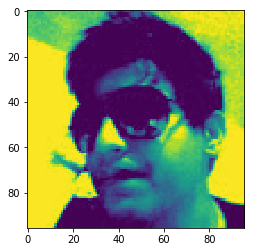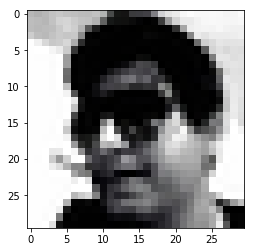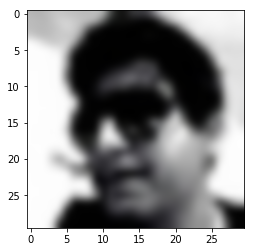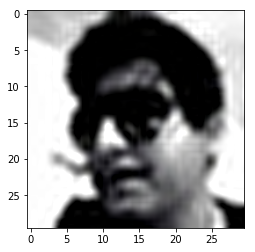Open In App
Related Articles
• Write an Interview Experience

# Python | Working with PNG Images using Matplotlib

Matplotlib is an amazing visualization library in Python for 2D plots of arrays. Matplotlib is a multi-platform data visualization library built on NumPy arrays and designed to work with the broader SciPy stack. It was introduced by John Hunter in the year 2002.
One of the greatest benefits of visualization is that it allows us visual access to huge amounts of data in easily digestible visuals. Matplotlib consists of several plots like line, bar, scatter, histogram etc.

In this article, we will see how can we work with PNG images using Matplotlib.

Code #1: Read a PNG image using Matplotlib

 `# importing pyplot and image from matplotlib``import` `matplotlib.pyplot as plt``import` `matplotlib.image as img`` ` `# reading png image file``im ``=` `img.imread(``'imR.png'``)`` ` `# show image``plt.imshow(im)`

Output:Code #2: Applying pseudocolor to image

Pseudocolor is useful for enhancing contrast of image.

 `# importing pyplot and image from matplotlib``import` `matplotlib.pyplot as plt``import` `matplotlib.image as img`` ` `# reading png image``im ``=` `img.imread(``'imR.png'``)`` ` `# applying pseudocolor ``# default value of colormap is used.``lum ``=` `im[:, :, ``0``]`` ` `# show image``plt.imshow(lum)`

Output:Code #3: We can provide another value to colormap with colorbar.

 `# importing pyplot and image from matplotlib``import` `matplotlib.pyplot as plt``import` `matplotlib.image as img`` ` `# reading png image``im ``=` `img.imread(``'imR.png'``)``lum ``=` `im[:, :, ``0``]`` ` `# setting colormap as hot``plt.imshow(lum, cmap ``=``'hot'``)``plt.colorbar()`

Output:Interpolation Schemes:
Interpolation calculates what the color or value of a pixel “should” be and this needed when we resize the image but want the same information. There’s missing space when you resize image because pixels are discrete and interpolation is how you fill that space.

Code # 4: Interpolation

 `# importing PIL and matplotlib``from` `PIL ``import` `Image ``import` `matplotlib.pyplot as plt`` ` `# reading png image  file``img ``=` `Image.``open``(``'imR.png'``)`` ` `# resizing the image``img.thumbnail((``50``, ``50``), Image.ANTIALIAS)``imgplot ``=` `plt.imshow(img)`

Output:Code #6: Here, ‘bicubic’ value is used for interpolation.

 `# importing pyplot from matplotlib``import` `matplotlib.pyplot as plt`` ` `# importing image from PIL``from` `PIL ``import` `Image `` ` `# reading image``img ``=` `Image.``open``(``'imR.png'``)`` ` `img.thumbnail((``30``, ``30``), Image.ANTIALIAS) `` ` `# bicubic used for interpolation``imgplot ``=` `plt.imshow(img, interpolation ``=``'bicubic'``)`

Output:Code #7: ‘sinc’ value is used for interpolation.

 `# importing PIL and matplotlib``from` `PIL ``import` `Image ``import` `matplotlib.pyplot as plt`` ` `# reading image``img ``=` `Image.``open``(``'imR.png'``)`` ` `img.thumbnail((``30``, ``30``), Image.ANTIALIAS)`` ` `# sinc used for interpolation``imgplot ``=` `plt.imshow(img, interpolation ``=``'sinc'``)`

Output:Reference: https://matplotlib.org/gallery/images_contours_and_fields/interpolation_methods.html